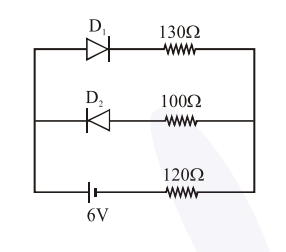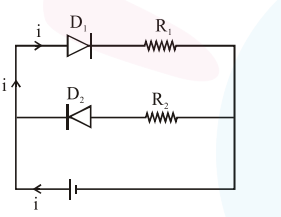# The circuit contains two diodes each with a forward resistance

Question:

The circuit contains two diodes each with a forward resistance of $50 \Omega$ and with infinite reverse resistance. If the battery voltage is $6 \mathrm{~V}$, the current through the $120 \Omega$ resistance is $\mathrm{mA}$.Solution:In this circuit $D_{1}$ will be forward bias and $D_{2}$ will be revers bias.

$\therefore$ There will be no current through $\mathrm{D}_{2}$ and $\mathrm{R}_{2}$

Apply KVL in circuit we get

$+6-50 \mathrm{i}-130 \mathrm{i}-120 \mathrm{i}=0$

$i=\frac{6}{300} \mathrm{~A}=\frac{6}{300} \times 1000 \mathrm{~mA}$

$\Rightarrow 20 \mathrm{~mA}$